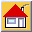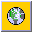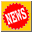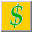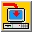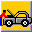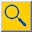Home Site Map About News Purchase Products Download Support Search

# Argus Open Numerical Environments' Functions

In Argus Open Numerical Environments, functions are calculation tools that you can use to perform value-returning, decision-making and action-taking operations automatically. Argus ONE provide a wide variety of functions that perform many different types of calculations.
Argus Open Numerical Environments has two types of functions: general functions and layer specific functions. You can use general functions in every layer type. For example, the general function Cos() returns the cosine of an angle; you can use this function in any layer type. Layer specific functions are available only in the appropriate layer. For example, the mesh layer function NumNodes(), returning the number of nodes in a mesh layer, has no meaning in non mesh type layers.
All of Argus Open Numerical Environments functions can be used both in layer and parameter expressions as well as in export templates.

Some functions which are not listed below can be found in the pages describing new features in version 2.5, 3 and 4.

Math Functions:

Abs(number)
Div(number,divisor)
Exp(number)
Log(number)
Log10(number)
Mod(number,divisor)
Power(number,exponent)
Sqrt(number)

Trigonometric and Hyperbolic Functions:

ACos(number)
ASin(number)
ATan(number)
ATan2(number,divisor)
Cos(number)
CosH(number)
Sin(number)
SinH(number)
Tan(number)
TanH(number)

String Functions:

Contains(string,substring)
Length(string)
Lower(string)
StrIndex(string,substring)
Substring(string,from,count)
Upper(string)

Spatial Functions:

X()
PositionX()
Y()
PositionY()

Logical Functions:

If(condition,true-val,false-val)
IsNA(expression)
IsNAN(expression)
IsNumber(expression)

Search Functions:

Index(selector,number-1,number-2,number-3,.....number-n)
Min(number-1,number-2,number-3,.....number-n)
Max(number-1,number-2,number-3,.....number-n)

Action Taking Functions:

Interpolate(expression)
DefaultValue(expression)

Mesh Layers Functions:

BandWidth([with_diagonals])
CountElements(condition)
CountNodes(condition)
MeshArea()
NumElements()
NumElemParameters()
NumNodeParameters()
NumNodes()
NumParameters()

Element Functions:

CountObjectsInElement(layer-name,contour-type)
ElementArea()
ElementIcon()
ElementName()
ElementNumber()
ElementOnBoundary()
GCenterX()
GCentery()
MinAngle()
NthAngle(angle-number)
NthNeighbourNum(neighbour-num)
NthNodeNum(node-num)
NthSideCenterX(side-num)
NthSideCenterY(side-num)
NthSideLength(side-num)
SumObjectsInElement(parameter-name,contour-type)
WSumObjectsInElement(parameter-name,contour-type)
NthNodeX(node_num)
NthNodeY(node_num)

Node Functions:

EffectiveArea()
NodeEffectiveLength(layer_name[,epsilon])
NodeIcon()
NodeName()
NodeNumber()
NodeAboveCntr(layer_name[,epsilon])
NodeOnBoundary()

Grid Layer Functions:

ActiveArea()
CountBlocks(condition)
NumColumns()
NumParameters()
NumRows()
TotalArea()
NthColumnPos(col-num)
NthRowPos(row-num)

Block Functions:

BlockArea()
BlockCenterX()
BlockCenterY()
BlockIcon()
BlockIsActive()
BlockOnBoundary()
Column()
CountObjectsInBlock(layer-name,contour-type)
NthBlockSideLength(h-v-side)
NumBlockParameters()
Row()
SumObjectsInBlock(parameter-name,contour-type)
WSumObjectsInBlock(parameter-name,contour-type)

GIS Functions:

ContourArea()
ContourLength()
CountObjectsInContour(layer_name,contour_type)
CountObjectsInElement(layer-name,contour-type)
NodeEffectiveLength(layer_name[,epsilon])
SumObjectsInElement(parameter-name,contour-type)
WSumObjectsInElement(parameter-name,contour-type)
NodeAboveCntr(layer_name[,epsilon])
CountObjectsInBlock(layer-name,contour-type)
SumObjectsInBlock(parameter-name,contour-type)
WSumObjectsInBlock(parameter-name,contour-type)
SumObjectsInContour(parameter_name,contour_type)
WSumObjectsInContour(parameter_name,contour_type)

Layer Functions:

LayerName()
NumParameters()
NthParamName(Parameter_Number)

Contour Functions:

ContourArea()
ContourIcon()
ContourLength()
ContourName()
CountObjectsInContour(layer_name,contour_type)
ContourType()
NthVertexX(number)
NthVertexY(number)
NumVertices()
SumObjectsInContour(parameter_name,contour_type)
WSumObjectsInContour(parameter_name,contour_type)

### Abs(number)

Description:
Returns the absolute value of number.

Example:
Abs(layer 1 - layer 2) returns the absolute of the difference in values between layer 1 and 2 at the point of evaluation.

### Div(number,divisor)

Description:
Returns the integer division INT(number/divisor) rounded towards zero.

Example:
Div(7,2) returns 3.00
Div(7,-2) returns -3.00
Div(-7,2) returns -3.00

### Exp(number)

Description:
Returns the natural exponent of number.

Example:
Exp(4) returns 54.5982

### Log(number)

Description:
Returns the natural logarithm (ln) of number.

Example:
Log(54.5982) returns 4.00

### Log10(number)

Description:
Returns the decimal logarithm (log) of number.

Example:
Log10(1000.00) returns 3.00

### Mod(number,divisor)

Description:
Returns the remainder (modulus) after number is divided by divisor. The result has the same sign as number.

Example:
Mod(7,2) returns 1.00
Mod(7,-2) returns 1.00
Mod(-7,2) returns -1.00

### Power(number,exponent)

Description:
Returns the value of number raised to exponent.

Example:
Power(7,2) returns 49.00

### Sqrt(number)

Description:
Returns the square root of number.

Example:
Sqrt(9) returns 3.00

### ACos(number)

Description:

Example:
ACos(-1) returns 3.14159

### ASin(number)

Description:

Example:
ASin(-1) returns -1.5708

### ATan(number)

Description:

Example:
ATan(412) returns 1.56837

### ATan2(number,divisor)

Description:

Example:
ATan2(2,0) returns 1.5708

### Cos(number)

Description:
Returns the cosine of number. Cycle: 2*Pi.

Example:
Cos(3.14) returns -0.99999

### CosH(number)

Description:
Returns the hyperbolic cosine of number.

Example:
CosH(4) returns 27.3082

### Sin(number)

Description:
Returns the sine of number. Cycle: 2*Pi.

Example:
Sin(1) returns 0.841471

### SinH(number)

Description:
Returns the hyperbolic sine of number.

Example:
SinH(3) returns 10.0179

### Tan(number)

Description:
Returns the tangent of number. number !> (2k+1)pi, Cycle: Pi

Example:
Tan(3.141592654) returns 4.10207e-10

### TanH(number)

Description:
Returns the hyperbolic tangent of number.

Example:
Tan(12) returns 1.00

### Contains(string,substring)

Description:
Returns True (1) if substring was found within string. Returns False (0) if substring was not found within string.

Example:
Contains("Argus","Arg") returns True (1) Contains("Argus","Args") returns False (0) Contains(ContourName(Sources),"Well") will return True (1) for all contours (in layer Sources) assigned a string containing the substring "Wells".

### Length(string)

Description:
Returns the number of characters in string (Integer)

Example:
Length("Argus") returns 5. Length("Argus"+"Numerical Environments") returns 27.

### Lower(string)

Description:
Returns string in lower case.

Example:
Lower("ARGUS") returns argus. Lower("Argus") returns argus. Lower("argus") returns argus.

### StrIndex(string,substring)

Description:
Returns the location (Integer) of the first character of substring within string. If substring is not present within string value returned is (0).

Example:
StrIndex("Argus","gus") returns 3. StrIndex("Argus","argus") returns 0.

### Substring(string,from,count)

Description:
Returns the string of characters of length count starting at (and including) from position in string. If from is smaller or larger than string, returns an empty string (""). If from is negative, it is counted from the length of string. If count is negative returns the string starting at from minus count.

Example:
Substring("Argus",3,3) returns the string "gus". Substring("Argus",-1,-2) returns the string "us". Substring("Argus",-1,2) returns the empty string "".

### Upper(string)

Description:
Returns string in upper case.

Example:
Upper("argus") returns ARGUS. Upper("Argus") returns ARGUS. Upper("argus") returns ARGUS. Upper(Lower("Argus")) returns ARGUS

### X()

Description:
Returns the X coordinate of the evaluation context.

Example:
X() in an element context, returns the X coordinate of the element center.

### PositionX()

Description:
Returns the X coordinate of the evaluation context.

Example:
Same as X()

### Y()

Description:
Returns the Y coordinate of the evaluation context.

Example:
Y() in an element context, returns the Y coordinate of the element center.

### PositionY()

Description:
Returns the Y coordinate of the evaluation context.

Example:
Same as Y()

### If(condition,true-val,false-val)

Description:
Returns true-val if condition evaluates to True and false-val if it evaluates to False. Any number of If functions can be nested as true-val and false-val arguments to construct more elaborate tests.

Example:
If(Formation1.Thickness<0,-1,Formation.Thickness) This function tests the thickness of a formation at each evaluation context, such as a node, and will return the value -1 for nodes at which the thickness is less than zero. At nodes the thickness is larger than or equal to zero, it returns the formation thickness.

### Index(selector,number-1,number-2,numbe-3,.....number-n)

Description:
Returns the value of an element in a series, selected by selector. Both selector and numbers-1, number-n can be any value returning expression.

Example:
Index(5,42.5,48.9,112.4,5.3,8.9,4.0,44.8) returns 8.9

### Min(number-1,number-2,number-3,.....number-n)

Description:
Returns the minimum value in a series. Numbers-1, number-n can be any value returning expression.

Example:
Min(1,2,3,4,5) returns 1

### Max(number-1,number-2,number-3,.....number-n)

Description:
Returns the maximum value in a series. Numbers-1, number-n can be any value returning expression.

Example:
Max(1,2,3,4,5) returns 5

### Interpolate(expression)

Description:
Interpolates all Information type layer parameters contained in the expression.

Example:
Interpolate(Rainfall*ElementArea) returns the interpolated value of the information layer "Rainfall" multiplied by the element area, at the evaluation context. In the above example the evaluation context is at the element center's coordinates.
Interpolate(Conductivity*Thickness) return the interpolated value of the "Conductivity" layer parameter multiplied by the interpolated value of the "Thickness" layer parameter at the evaluation context.
An expression can hold as many information layer parameters as needed, all parameters' values will be interpolated before the expression is evaluated.

### DefaultValue(expression)

Description:
Returns the default value of all Information type layer parameters contained in the expression.

Example:
DefaultValue(Rainfall*Concentration) returns the default value of the information layer parameter "Rainfall" multiplied by the default value of the information layer parameter "Concentration" disregarding any value assigned to contours in these layers (the default value of a parameter is set in the Layers Dialog. An expression can hold as many information layer parameters as needed, all parameters' values will be set to default before the expression is evaluated.

### IsNA(expression)

Description:
Returns True (1) if expression evaluates to \$n/a and False (0) if expression does not evaluate to \$n/a.

Example:
isNA(if(NodeOnBoundary()|NodeAboveCntr(BCType)=1,BCType,\$n/a)). The function isNA returns:
True (1) if the if statement evaluates to false and returns \$n/a, and False (0) if the if statement evaluates to true and returns BCType which is not equal to \$n/a.
This is useful for creating Export Template Script which will export only nodes with boundary conditions values.

### IsNAN(expression)

Description:
Returns True (1) if expression evaluates to \$NaN and False (0) if expression does not evaluate to \$NaN.

### IsNumber(expression)

Description:
Returns True (1) if expression evaluates to a number and False (0) if expression evaluates to \$n/a or \$NaN.

Example:
These two functions can be used to test expressions returning illegal numbers when evaluated. Nest any expression within one of these functions and search the mesh or grid for nodes, elements or blocks that are NaN or Numbers.

### BandWidth([with_diagonals])

Description:
Returns the half-bandwidth of a mesh. If the optional argument with_diagonals is used, returns the half-bandwidth including diagonals in a Quadrilateral mesh. The argument with_diagonals is not used for Triangular meshes. If more than one continuous mesh is present in a mesh layer, returns the largest half-bandwidth of all meshes.

Example:
Some models require that you provide the bandwidth as part of their input. This may serve the model to dimension arrays and matrices. Use this function in an export template to provide the model with the information required. For instance, if the model requires the full bandwidth create the expression: Bandwidth()*2+1

### CountElements(condition)

Description:
Returns the number of elements in a mesh layer which satisfy the condition.

Example:
Some models require that you provide the number of elements satisfying a condition. These may be the number of elements in which you specify boundary conditions. Use this function in an export template to provide the model with the information required. For instance, if the model requires the number of elements through which rivers are flowing, create the expression: CountElements(CountObjectsInElement(River,1)!=0)

### CountNodes(condition)

Description:
Returns the number of nodes in a mesh layer which satisfy the condition.

Example:
Some models require that you provide the number of nodes satisfying a condition. These may be the number of nodes in which you specify boundary conditions. Use this function in an export template to provide the model with the information required. For instance, if the model requires the number of nodes acting as sources, create the expression: CountNodes(NodeAboveCntr(Wells,0))

### MeshArea()

Description:
Returns the total area of the elements in a mesh layer. If the mesh layer contains more than one mesh, or some disjoined elements, this function returns the total area of all the elements.

### NumElements()

Description:
Returns the total number of elements in a mesh layer. If the mesh layer contains more than one mesh, or some disjoined elements, this function returns the total number of elements in the layer.

note:
Used mainly in Export templates to loop over the number of elements.

### NumElemParameters()

Description:
Returns the number of element type parameters of a mesh layer.

note:
Used mainly in Export templates to loop over the number of element related parameters.

### NumNodeParameters()

Description:
Returns the number of node type parameters of a mesh layer.

note:
Used mainly in Export templates to loop over the number of node related parameters.

### NumNodes()

Description:
Returns the total number of nodes in a mesh layer. If the mesh contains more than one mesh, or some disjoined elements, this function returns the total number of nodes in the layer.

note:
Used mainly in Export templates to loop over the number of nodes.

### NumParameters()

Description:
Returns the number of mesh layer related parameters.

note:
Used mainly in Export templates to loop over the number of mesh layer related parameters.

### CountObjectsInElement(layer-name,contour-type)

Description:
Returns the number of objects of type contour-type in layer layer-name, intersecting or contained in the element. If contour-type is omitted, returns the total number of all objects intersecting or contained in the element.

Example:
The function CountObjectsInElement(Domain Layer) counts the number of objects in the layer "Domain Layer" intersecting or contained by the element and returns: 0 - if the element is not intersected by, or contains no objects. 1 - if the element is intersected by, or contains one object of any type. N - if the element is intersected by, or contains N objects of any type. Example: The function CountObjectsInElement(Domain Layer,1) counts the number of open contours in the layer "Domain Layer" intersecting or contained by the element and returns: 0 - if the element is not intersected by, or contains no open contours. 1 - if the element is intersected by, or contains one open contour. N - if the element is intersected by, or contains N open contours.

### ElementArea()

Description:
Returns the area of the element.

Example:
(Rainfall*ElementArea()) if rainfall is an information layer describing the rainfall distribution, returns the replenishment rate (mass) contributed by the element.

### ElementIcon()

Description:
Returns the index (integer) of the icon assigned to the element. If the element is not assigned an icon, returns 0 (zero). Icons are indexed from 1, according to their order of appearance in the Element Icon pop-up menu.

### ElementName()

Description:
Returns the name (string) assigned to the element. If the element is not assigned a name, returns an empty string. The return value is case sensitive.

### ElementNumber()

Description:
Returns the number of the element.

Note:
Used mainly in Export templates to export the element number.

### ElementOnBoundary()

Description:
Returns True (1) if one or more of the element's nodes lie on the perimeter of the domain occupied by elements, otherwise returns False (0).

Example:
ElementOnBoundary() returns 1 if the element is a boundary element, otherwise returns 0. Can be used in an Export template using the export template If statement to export only boundary elements.

### GCenterX()

Description:
Returns the X coordinate of the element's center of gravity.

### GCenterY()

Description:
Returns the Y coordinate of the element's center of gravity.

### MinAngle()

Description:
Returns the element's minimal angle in degrees.

Example:
Create an element parameter and assign it the MinAngle() function. Use the color palette to view the distribution of elements by minimum angle.

### NthAngle(angle-number)

Description:
Returns the element's angle-number angle in degrees.

Example:
NthAngle(1) returns the value of the elements' first angle corresponding with the element's first node.

### NthNeighbourNum(neighbour-num)

Description:
Returns the number of the element's neighbour-num neighboring element. Returns 0 if there is no such element. Returns zero for all three arguments if the element is disjoined.

### NthNodeNum(node-num)

Description:
Returns the node number of the element's node-num.

Note:
Used mainly in Export templates to export the element's node numbers.

### NthNodeX(node_num)

Description:
Returns the X position of the element's node_num node.

### NthNodeY(node_num)

Description:
Returns the Y position of the element's node_num node. New Node Functions

### NthSideCenterX(side-num)

Description:
Returns the X coordinate of the element's side-num side midpoint.

### NthSideCenterY(side-num)

Description:
Returns the Y coordinate of the element's side-num side midpoint.

### NthSideLength(side-num)

Description:
Returns the length of the element's side-num side.

### SumObjectsInElement(parameter-name,contour-type)

Description:
Returns the sum of the objects' values of type contour-type in parameter parameter-name, intersecting or contained in the element. If contour-type is omitted, returns the sum of values of all objects intersecting or contained in the element.

Example:
The function SumObjectsInElement(Well Layer,0) can be used to sum the values of the specified variable at the point objects which are in the layer "Well Layer" and that are contained by the element. If divided by CountObjectsInElement(Well Layer,0) it shall return the average value of the specified function.

### WSumObjectsInElement(parameter-name,contour-type)

Description:
Returns the weighted sum of objects' values of type contour-type in parameter parameter-name, intersecting or contained in the element. If contour-type is omitted, returns the weighted sum of values of all objects intersecting or contained in the element.

Example:
The function WSumObjectsInElement(Fractures Layer,1) computes the product of each intersecting or contained segment length by its value and sums them.

### NthNodeX(node_num)

Description:
Returns the X position of the element's node_num node.

### NthNodeY(node_num)

Description:
Returns the Y position of the element's node_num node. New Node Functions

### EffectiveArea()

Description:
Returns the node's effective area. Node effective area is defined as the sum of 1/element-nodes-number of the area of each of the elements connected to the node.

Example:
(Rainfall*EffectiveArea()) if rainfall is an information layer describing the rainfall distribution, returns the replenishment rate (mass) contributed by the node.

### NodeEffectiveLength(layer_name[,epsilon])

Description:
Returns half of the length of the two segments connected to the node which lies above the contour object. If the node is the first or the last node above an open contour, it returns the half length of only the last segment.

Example:
NodeEffectiveLength(Rivers) returns half the length of segments connected to the node which also lies above the open contour object in Rivers layer. For middle nodes, it returns half the length of both segments connected to it and which also lie above the object. For an end node, it returns half the length of the single segment connected to it and which also lies above the object. Can be used for boundary conditions. For instance, to calculate the mass contributed to a node from a river or a fracture, create the following node parameter expression: Rivers*NodeEffectiveLength(Rivers)

### NodeIcon()

Description:
Returns the index (integer) of the icon assigned to the node. If the node is not assigned an icon, returns 0 (zero). Icons are indexed from 1, according to their order of appearance in the Node Icon pop-up menu.

### NodeName()

Description:
Returns the name (string) assigned to the node. If the node is not assigned a name, returns an empty string. The return value is case sensitive.

### NodeNumber()

Description:
Returns the number of the node.

Note:
Used mainly in Export templates to export the node's number.

### NodeAboveCntr(layer_name[,epsilon])

Description:
Returns the type of object the node lies above or within layer-name:
0 - If the node does not lie above or within any contour.
1 - If the node lies above a point object.
2 - If the node lies above an open contour.
3 - If the node lies above a close contour.
4 - If the node lies inside a close contour.

Example:
The function if(NodeAboveCntr(BC)=1,BC,DefaultValue(BC)) returns the value of the boundary condition assigned to the point object that lies below a node and if the node does not lie above a point object, the default value of the BC layer. This is useful for allocating boundary conditions types and values to node elements.

### NodeOnBoundary()

Description:
Returns True (1) if the node lies on the perimeter of the domain occupied by elements the node belongs to, otherwise returns False (0).

Note:
Can be used in an export template using the export template script If statement to export only boundary nodes.

### ActiveArea()

Description:
Returns the total area of active blocks in the current grid layer.

### CountBlocks(condition)

Description:
Returns the number of blocks in a grid layer which satisfy the condition.

Example:
Some models require that you provide the number of blocks satisfying a condition. These may be the number of blocks in which you specify boundary conditions. Use this function in an export template to provide the model with the information required. For instance, if the model requires the number of blocks through which rivers are flowing, create the expression: CountBlocks(CountObjectsInBlock(River,1)!=0)

### NumColumns()

Description:
Returns the number of grid columns.

Note:
Used mainly in Export templates to loop over the number of grid columns.

### NumParameters()

Description:
Returns the number of grid related layer parameters.

Note:
Used mainly in Export templates to loop over the number of grid layer related parameters.

### NumRows()

Description:
Returns the number of grid rows.

Note:
Used mainly in Export templates to loop over the number of grid rows.

### TotalArea()

Description:
Returns the total grid area.

Example:
The expression (TotalArea()-ActiveArea()) returns the area of all inactive blocks in a grid.

### NthColumnPos(col-num)

Description:
Returns col-num's grid line coordinate. When in "grid centered" grid, this coordinate is identical to the block center's X coordinate. When in "block centered" grid, this coordinate is the minimum X coordinate of col-num (or block) depending on the coordinate system direction.

Example:
Used mainly in grid Export templates to export grid lines coordinates.

### NthRowPos(row-num)

Description:
Returns row-num's grid line coordinate. When in "grid centered" grid, this coordinate is identical to the block center's Y coordinate. When in "block centered" grid, this coordinate is the minimum Y coordinate of row-num (or block) depending on the coordinate system direction.

Example:
Used mainly in grid Export templates to export grid lines coordinates.

### BlockArea()

Description:
Returns the block's area.

Example:
(Concentration*BlockArea()) if Concentration is an information layer describing the contaminant concentration distribution, the expression returns the contaminant mass contributed by the block.

### BlockCenterX()

Description:
Returns the block's center X coordinate.

### BlockCenterY()

Description:
Returns the block's center Y coordinate.

### BlockIcon()

Description:
Returns the index (integer) of the icon assigned to a block. If the block is not assigned an icon, returns 0 (zero). Icons are indexed from 1, according to their order of appearance in the Block Icon pop-up menu.

### BlockIsActive()

Description:
Returns True (1) if block is active, otherwise returns False (0).

### BlockOnBoundary()

Description:
Returns True (1) if block has at least one side on the grid edge, or block is inactive. Otherwise returns False (0).

### Column()

Description:
Returns the column number the block belongs to.

### CountObjectsInBlock(layer-name,contour-type)

Description:
Returns the number of objects of type contour-type in layer layer-name, intersecting or contained in the block. If contour-type is omitted, returns the total number of all objects intersecting or contained in the block.

Example:
The function CountObjectsInBlock(Domain Layer) counts the number of objects in the layer "Domain Layer" intersecting or contained by the block and returns:
0 - if the block is not intersected by, or contains no objects.
1 - if the block is intersected by, or contains one object of any type.
N - if the block is intersected by, or contains N objects of any type.
Example: The function CountObjectsInBlock(Domain Layer,1) counts the number of open contours in the layer "Domain Layer" intersecting or contained by the block and returns:
0 - if the block is not intersected by, or contains no open contours.
1 - if the block is intersected by, or contains one open contour.
N - if the block is intersected by, or contains N open contours.

### NthBlockSideLength(h-v-side)

Description:
Returns the h-v-side length of the block.

Example:
NthBlockSideLength(1) returns the block's vertical length.
NthBlockSideLength(2) returns the block's horizontal length.

### NumBlockParameters()

Description:
Returns the number of block and grid related layer parameters.

Example:
Example: Used mainly in Export templates to loop over the number of block layer related parameters.

### Row()

Description:
Returns the row number the block belongs to.

### SumObjectsInBlock(parameter-name,contour-type)

Description:
Returns the sum of the objects' values of type contour-type in parameter parameter-name, intersecting or contained in the block. If contour-type is omitted, returns the sum of values of all objects intersecting or contained in the block.

### WSumObjectsInBlock(parameter-name,contour-type)

Description:
Returns the weighted sum of objects' values of type contour-type in parameter parameter-name, intersecting or contained in the block. If contour-type is omitted, returns the weighted sum of values of all objects intersecting or contained in the block.

Example:
The function WSumObjectsInBlock(Fractures Layer,1) computes the product of each intersecting or contained segment length by its value and sums them.

### LayerName()

Description:
Returns the name (string) of the layer. The return value is case sensitive.

### NumParameters()

Description:
Returns the number of parameters assigned to the layer. Previously this function was only available for mesh and grid layers. It is now available for all layer types.

### NthParamName(Parameter_Number)

Description:
Returns the name (string) of the Parameter_Number layer parameter. The return value is case sensitive.

### ContourArea()

Description:
Returns the area (real) of a contour. If the contour is an open or point contour the value returned is zero (0).

### ContourIcon()

Description:
Returns the index (integer) of the icon assigned to the contour. If the contour is not assigned an icon, returns 0 (zero). Icons are indexed from 1, according to their order of appearance in the Contour Icon pop-up menu.

### ContourLength()

Description:
Returns the length (real) of a contour.

Example:
If an open contour object represents a line boundary condition such as a river, a fault or just a line boundary, and the mass contributed by the object is given as the total mass (and not mass per unit length) then the mass per unit length can be calculated by the expression: Rivers.Mass/ContourLength() If the information layer contains point objects as well, the following expression prevents accidental division by zero: Rivers.Mass/If(ContourType=1,ContourLength(),1) This function can also be used to calculate a quantity per unit length on closed contours representing for instance, internal boundaries.

### ContourName()

Description:
Returns the name (string) assigned to the contour. If the contour is not assigned a name, returns an empty string. The return value is case sensitive.

### CountObjectsInContour(layer_name,contour_type)

Description:
Returns the number (integer) of objects of type contour_type in layer layer_name, intersecting or contained in the contour. If contour_type is omitted, returns the total number of all objects intersecting or contained in the contour.

Example:
The function CountObjectsInContour(Domain Layer) counts the number of objects in the layer "Domain Layer" intersecting or contained by the contour and returns: 0 - if the contour is not intersected by, or does not contain any object. 1 - if the contour is intersected by, or contains one object of any type. N - if the contour is intersected by, or contains N objects of any type.

### ContourType()

Description:
Returns the type of the contour: 1 for a point contour 2 for an open contour 3 for a close contour

### NthVertexX(number)

Description:
Returns the X coordinate of the contour's number vertex.

### NthVertexY(number)

Description:
Returns the Y coordinate of the contour's number vertex.

### NumVertices()

Description:
Returns a contour's number of vertices.

### SumObjectsInContour(parameter_name,contour_type)

Description:
Returns the sum of the objects' values of type contour_type in parameter parameter_name, intersecting or contained in the contour. If contour_type is omitted, returns the sum of values of all objects intersecting or contained in the contour.

Example:
The function SumObjectsInContour(Wells Layer,0) can be used to sum the values of the specified variable of the point objects which are in the layer "Wells Layer" and that are contained by the contour. If divided by CountObjectsInContour(Wells Layer,0) it shall return the average value of the specified function.

### WSumObjectsInContour(parameter_name,contour_type)

Description:
Returns the weighted sum of objects' values of type contour_type in parameter parameter_name, intersecting or contained in the contour. If contour_type is omitted, returns the weighted sum of values of all objects intersecting or contained in the contour.

Example:
The function WSumObjectsInContour(Fractures Layer,1) computes the product of each intersecting or contained segment length by its value and sums them.

To find out more about Argus and its products email marketing@argusone.com or call:
+972-9-957-5752
Tech. support: support@argusone.com
snail mail: Argus Holdings, POB 6254, Herzelia, 46160, Israel